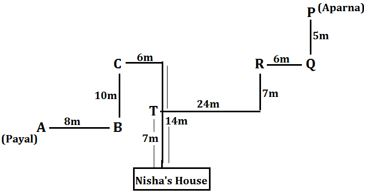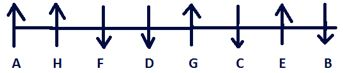Latest Banking jobs   »

# Reasoning Ability Quiz For FCI Phase I 2022- 17th September

Directions (1-5): Study the following information carefully and answer the questions given below:
Payal starts walking from point A, walks 8m in east direction and reach at point B. From point B she takes a left turn and walks 10m to reach at point C, then takes two consecutive right turn and walks 6m and 14m respectively to reach her friend Nisha’s house. Aparna starts walking in south direction from point P and walks 5m to reach at point Q, then takes a right turn and walks 6m to reach at point R. From point R she takes a left turn and walks 7m to reach at point S. From point S she takes a right turn and walks 24m and reach at point T, then finally takes a left turn and walks 7m to reach her friend Nisha’s house.

Q1. What is the distance between point C to point R?
(a) 12m
(b) 28m
(c) 30m
(d) 20m
(e) None of these

Q2. What is the shortest distance between point S to Nisha’s house?
(a) 49m
(b) 25m
(c) 16m
(d) 30m
(e) None of these

Q3. What is the direction of point P with respect to point B?
(a) South-West
(b) North
(c) East
(d) North-East
(e) None of these

Q4. What is the direction and distance between point T with respect to point B?
(a) 3√5m, south-west
(b) 45m, east
(c) 3√5m, north-east
(d) 35, west
(e) None of these

Q5. What is the direction of point T with respect to point P?
(a) South
(b) South-West
(c) North-East
(d) West
(e) None of these

Q6. Which of the following symbols should replace the question mark in the given expression to make A ≥ D definitely true?
A= G ≥ B ? F ? M = D
(a) >, >
(b) =, >
(c) ≤, ≤
(d) ≤, <
(e) None of these

Q7. Which of the following symbols should replace the question mark(?) in the given expression in order to make the expressions A < B definitely true and D ≤ A definitely true?
B ? E = A ? F ≥ N = D
(a) >, ≤
(b) =, >
(c) <, >
(d) >, =
(e) ≤, <

Q8. Which of the following symbols should replace the question mark in the given expression to make N ≥ D definitely true?
A= G ≥ B, N ? F ? M = D, B > N
(a) >, ≥
(b) =, >
(c) ≤, ≤
(d) ≤, <
(e) None of these

Q9. Which of the following symbols should replace the question mark(?) in the given expression in order to make the expressions M < E definitely false and B ≤ A definitely true?
M ? B ? E = A
(a) >, ≤
(b) =, >
(c) <, >
(d) =, =
(e) ≤, <

Q10. Which of the following symbols should replace the question mark in the given expression to make G ≥ F and M <B definitely true?
A? G ? B ? F ? M
(a) >, >,>, ≤
(b) =, >,>, ≤
(c) ≤, ≤,>, ≤
(d) ≤, <,>, ≤
(e) None of these

Direction (11-15): Study the given information carefully and answer the questions that follow:
There are eight friends A, B, C, D, E, F, G and H who are sitting in a row such that some are facing in the south direction and some are facing north direction. F and D face the same direction as B is facing. D sits third to the right of A. C and E are immediate neighbours but both face opposite directions with respect to each other. A sits at one of the corner. H sits fourth to the right of C. More than three persons sit between B and F. G sits third right of H. C is neither immediate neighbour of A nor D. E does not sit at any end. F sits immediate right of D. G is facing same direction as E who does not face south.

Q11. Who among the following sits immediate right of H?
(a) G
(b) C
(c) E
(d) F
(e)None of these

Q12. How many persons sit between D and E?
(a) Four
(b) One
(c) Three
(d) Two
(e) None

Q13. Who among the following sit immediate left of the one who sits third right of G?
(a) H
(b) F
(c) A
(d) E
(e) No one

Q14. What is the position of A with respect of F?
(a) Immediate right
(b) Third to the left
(c) Second to the right
(d) Immediate left
(e) None of these

Q15. Who among the following sits second right of B?
(a) G
(b) A
(c) F
(d) C
(e) None of these

Solutions

Solutions (1-5):
Sol.S1. Ans. (c)
S2. Ans. (b)
S3. Ans. (d)
S4. Ans. (c)
S5. Ans. (b)

S6. Ans. (e)
S7. Ans. (d)
S8. Ans. (e)
S9. Ans. (a)
S10. Ans. (e)

Solutions (11-15):
Sol.S11. Ans. (d)
S12. Ans. (d)
S13. Ans. (e)
S14. Ans. (c)
S15. Ans. (d)#### Congratulations!Download Hindu Review of October 2021: Free PDF